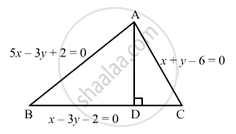Advertisement Remove all ads

# Three Sides Ab, Bc and Ca of a Triangle Abc Are 5x − 3y + 2 = 0, X − 3y − 2 = 0 and X + Y − 6 = 0 Respectively. Find the Equation of the Altitude Through the Vertex A. - Mathematics

Answer in Brief

Three sides AB, BC and CA of a triangle ABC are 5x − 3y + 2 = 0, x − 3y − 2 = 0 and x + y − 6 = 0 respectively. Find the equation of the altitude through the vertex A.

Advertisement Remove all ads

#### Solution

The sides ABBC and CA of a triangle ABC are as follows:
5x − 3y + 2 = 0                     ... (1)
x − 3y − 2 = 0                       ... (2)
x + y − 6 = 0                         ... (3)
Solving (1) and (3):
x = 2 , y = 4Thus, AB and CA intersect at A (2, 4).
Let AD be the altitude.

$AD \perp BC$

$\therefore$ Slope of AD $\times$ Slope of BC = −1
Here, slope of BC = slope of the line (2) =  $\frac{1}{3}$

$\therefore \text { Slope of AD }\times \frac{1}{3} = - 1$

$\Rightarrow \text { Slope of AD } = - 3$

Hence, the equation of the altitude AD passing through A (2, 4) and having slope −3 is

$y - 4 = - 3\left( x - 2 \right)$

$\Rightarrow 3x + y = 10$

Concept: Straight Lines - Equation of Family of Lines Passing Through the Point of Intersection of Two Lines
Is there an error in this question or solution?
Advertisement Remove all ads

#### APPEARS IN

RD Sharma Class 11 Mathematics Textbook
Chapter 23 The straight lines
Exercise 23.1 | Q 12 | Page 78
Advertisement Remove all ads
Advertisement Remove all ads
Share
Notifications

View all notifications

Forgot password?